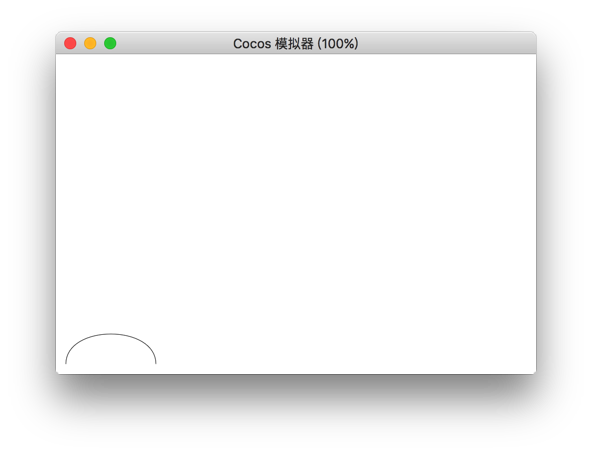# QuadraticCurveTo

The `quadraticCurveTo()` method adds a point to the current path by using the specified control point that represents the Quadratic Bezier curve. Tip: The Quadratic Bezier curve requires two points. The first point is for the control point in the second Bessel calculation, and the second point is the end point of the curve. The starting point of the curve is the last point in the current path

Parameter Description
cpx The x coordinate of the Bezier control point
cpy The y coordinate of the Bezier control point
x The x coordinate of the end point
y The y coordinate of the end point

## Example

``````var ctx = node.getComponent(cc.Graphics);
ctx.moveTo(20,20);
ctx.quadraticCurveTo(20,100,200,20);
ctx.fill();
``````Return to Graphics Component Reference.